# MCQ in Differential Calculus (Limits and Derivatives) Part 2| ECE Board Exam

(Last Updated On: February 5, 2020)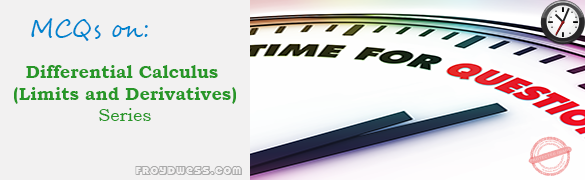This is the Multiple Choice Questions Part 2 of the Series in Differential Calculus (Limits and Derivatives) topic in Engineering Mathematics. In Preparation for the ECE Board Exam make sure to expose yourself and familiarize in each and every questions compiled here taken from various sources including but not limited to past Board Examination Questions in Engineering Mathematics, Mathematics Books, Journals and other Mathematics References.

### Multiple Choice Questions Topic Outline

• MCQ in Derivatives | MCQ in Derivatives of Algebraic functions | MCQ in Derivatives of Exponential functions | MCQ in Derivatives of Logarithmic functions | MCQs in Derivatives of Trigonometric functions | MCQ in Derivatives of Inverse Trigonometric functions | MCQ in Derivatives of Hyperbolic functions

#### Continue Practice Exam Test Questions Part II of the Series

Choose the letter of the best answer in each questions.

• A. 0
• B. 1
• C. 2
• D. 3

52. Simplify the expression: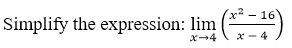• A. 1
• B. 8
• C. 0
• D. 16

53. Evaluate the following limit: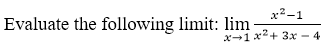• A. 2/5
• B. infinity
• C. 0
• D. 5/2

54. Evaluate the limit ( x – 4 ) / (x2 – x – 12) as x approaches 4.

• A. 0
• B. undefined
• C. 1/7
• D. infinity

55. Evaluate the limit (1n x ) / x as x approaches positive infinity.

• A. 1
• B. 0
• C. e
• D. infinity

56. Evaluate the following limit: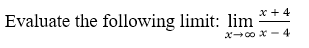• A. 1
• B. Indefinite
• C. 0
• D. 2
• A. 0
• B. ½
• C. 2
• D. -1/2

58. Evaluate the following: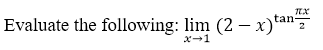• A. Infinity
• B. eπ
• C. 0
• D. e2/π

59. Find dy/dx if y = 52x-1

• A. 52x-1 ln 5
• B. 52x-1 ln 25
• C. 52x-1 ln 10
• D. 52x-1 ln 2

60. Find dy/dx if y = e√x

• A. e√x  / 2√x
• B. e√x / √x
• C. ex  / √x
• D. e√x – 2√x

61. Find dy/dx if y = x2 + 3x + 1 and x = t2 + 2.

• A. 4t3 + 14t2
• B. t3 + 4t
• C. 4t3 + 14t
• D. 4t3 + t

62. Evaluate the first derivative of the implicit function: 4×2 + 2xy + y2 = 0

• A. (4x + y) / (x + y)
• B. –[(4x + y) / (x + y)]
• C. (4x – y) / (x + y)
• D. –[(4x + y) / (x –y)]

63. Find the derivative of (x + 5) / (x2 – 1) with respect to x.

• A. DF(x) = (-x2 – 10x – 1) / (x2 – 1)2
• B. DF(x) = (x2 + 10x – 1) / (x2 – 1)2
• C. DF(x) = (x2 –10x – 1) / (x2 – 1)2
• D. DF(x) = (-x2 –10x + 1) / (x2 – 1)2

64. If a simple constant, what is the derivative of y = xa?

• A. a xa-1
• B. (a – 1)x
• C. xa-1
• D. ax

65. Find the derivative of the function 2×2 + 8x + 9 with respect to x.

• A. Df(x) = 4x – 8
• B. Df(x) = 2x + 9
• C. Df(x) = 2x + 8
• D. Df(x) = 4x + 8

66. What is the first derivative dy/dx of the expression (xy)x = e?

• A. – y(1 + ln xy) / x
• B. 0
• C. – y(1 – ln xy) / x2
• D. y/x

67. find the derivative of (x + 1)3 / x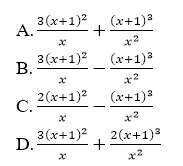68. Given the equation: y = (e ln x)2, find y’.

• A. ln x
• B. 2 (ln x) / x
• C. 2x
• D. 2 e ln x

69. Find the derivatives with respect to x of the function √(2 – 3×2)

• A. -2×2 / √(2 – 3×2)
• B. -3x / √(2 – 3×2)
• C. -2×2 / √(2 + 3×2)
• D. -3x / √(2 + 3×2)

70. Differentiate ax2 + b to the ½ power.

• A. -2ax
• B. 2ax
• C. 2ax + b
• D. ax + 2b

71. Find dy/dx if y = ln √x

• A. √x / ln x
• B. x / ln x
• C. 1 / 2x
• D. 2 / x

72. Evaluate the differential of tan Ѳ.

• A. ln sec Ѳ dѲ
• B. ln cos Ѳ dѲ
• C. sec Ѳ tan Ѳ dѲ
• D. sec2 Ѳ dѲ

73. If y = cos x, what is dy/dx?

• A. sec x
• B. –sec x
• C. sin x
• D. –sin x

74. Find dy/dx: y = sin (ln x2).

• A. 2 cos (ln x2)
• B. 2 cos (ln x2) / x
• C. 2x cos (ln x2)
• D. 2 cos (ln x2) / x2

75. The derivative of ln (cos x) is:

• A. sec x
• B. –sec x
• C. –tan x
• D. tan x

76. Find the derivative of arcos 4x with respect to x.

• A. -4 / [1 – (4x)^2]^2
• B. -4 / [1 – (4x)]^0.5
• C. 4 / [1 – (4x)^2]^0.5
• D. -4 / [(4x)^2 – 1]^0.5

77. What is the first derivative of y = arcsin 3x.

• A. –[3 / (1 + 9×2)]
• B. 3 / (1 + 9×2)
• C. –[3 / √(1 – 9×2)]
• D. 3 / √(1 – 9×2)

78. If y = x (ln x), find d2y / dx2.

• A. 1 / x2
• B. -1 / x
• C. 1 / x
• D. -1 / x2

79. Find the second derivative of y = x-2 at x = 2.

• A. 96
• B. 0.375
• C. -0.25
• D. -0.875

80. Given the function f(x) = x3 – 5x + 2, find the value of the first derivative at x = 2, f’ (2).

• A. 7
• B. 3×2 – 5
• C. 2
• D. 8

81. Given the function f(x) = x to the 3rd power – 6x + 2, find the value of the first derivative at x = 2, f’(2)

• A. 6
• B. 3×2 – 5
• C. 7
• D. 8

82. Find the partial derivatives with respect to x of the function: xy2 – 5y + 6.

• A. y2 – 5
• B. xy – 5y
• C. y2
• D. 2xy

83. Find the point in the parabola y2 = 4x at which the rate of change of the ordinate and abscissa are equal.

• A. (1, 2)
• B. (2, 1)
• C. (4, 4)
• D. (-1, 4)

84. Find the slope of the line tangent to the curve y = x3 – 2x + 1 at x = 1.

• A. 1
• B. ½
• C. 1/3
• D. ¼

85. Determine the slope of the curve x2 + y2 – 6x – 4y – 21 = 0 at (0, 7).

• A. 3/5
• B. -2/5
• C. -3/5
• D. 2/5

86. Find the slope of the tangent to a parabola y = x2 at a point on the curve where x = ½.

• A. 0
• B. 1
• C. ¼
• D. -1/2

87. Find the slope of the ellipse x2 + 4y2 – 10x + 16y + 5 = 0 at the point where y = -2 + 80.5 and x = 7.

• A. -0.1654
• B. -0.1538
• C. -0.1768
• D. -0.1463

88. Find the slope of the tangent to the curve y = x4 – 2×2 + 8 through point (2, 16).

• A. 20
• B. 1/24
• C. 24
• D. 1/20

89. Find the slope of the tangent to the curve y2 = 3×2 + 4 through point (-2, 4)

• A. -3/2
• B. 3/2
• C. 2/3
• D. -2/3

90. Find the slope of the line whose parametric equations are x = 4t + 6 and y = t – 1.

• A. -4
• B. ¼
• C. 4
• D. -1/4

91. What is the slope of the curve x2 + y2 – 6x + 10y + 5 = 0 at (1, 0).

• A. 2/5
• B. 5/2
• C. -2/5
• D. -5/2

92. Find the slope of the curve y = 6(4 + x) ½ at (0, 12).

• A. 0.67
• B. 1.5
• C. 1.33
• D. 0.75

93. Find the acute angle that the curve y = 1 – 3×2 cut the x-axis.

• A. 77°
• B. 75°
• C. 79°
• D. 120°

94. Find the angle that the line 2y – 9x – 18 = 0 makes with the x-axis.

• A. 74.77°
• B. 4.5°
• C. 47.77°
• D. 77.47°

95. Find the equation of the tangent to the curve y = x + 2×1/3 through point (8, 12)

• A. 7x – 6y + 14 = 0
• B. 8x + 5y + 21 = 0
• C. 5x – 6y – 15 = 0
• D. 3x – 2y – 1 = 0

96. What is the radius of curvature at point (1, 2) of the curve 4x – y2 = 0?

• A. 6.21
• B. 5.21
• C. 5.66
• D. 6.66

97. Find the radius of curvature at any point of the curve y + ln (cos x) = 0.

• A. cos x
• B. 1.5707
• C. sec x
• D. 1

98. Determine the radius of curvature at (4, 4) of the curve y2 – 4x = 0.

• A. 24.4
• B. 25.4
• C. 23.4
• D. 22.4

99. Find the radius of curvature of the curve x = y3 at (1, 1)

• A. 4.72
• B. 3.28
• C. 4.67
• D. 5.27

100. The chords of the ellipse 64×2 + 25y2 = 1600 having equal slopes of 1/5 are bisected by its diameter. Determine the equation of the diameter of the ellipse.

• A. 5x – 64y = 0
• B. 64x – 5y = 0
• C. 5x + 64y = 0
• D. 64x + 5y = 0

#### Online Questions and Answers in Differential Calculus (Limits and Derivatives) Series

Following is the list of multiple choice questions in this brand new series:

Differential Calculus (Limits and Derivatives) MCQ
PART 1: MCQ from Number 1 – 50                        Answer key: PART I
PART 2: MCQs from Number 51 – 100                   Answer key: PART II

P inoyBIX educates thousands of reviewers and students a day in preparation for their board examinations. Also provides professionals with materials for their lectures and practice exams. Help me go forward with the same spirit.

“Will you subscribe today via YOUTUBE?”

Subscribe

PinoyBIX Engineering. © 2014-2020 All Rights Reserved | How to Donate? |